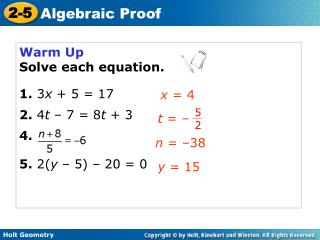DownloadDownload PresentationWarm Up Solve each equation. 1. 3 x + 5 = 17 2. 4 t – 7 = 8 t + 3 4. 5. 2( y – 5) – 20 = 0

# Warm Up Solve each equation. 1. 3 x + 5 = 17 2. 4 t – 7 = 8 t + 3 4. 5. 2( y – 5) – 20 = 0

Télécharger la présentation## Warm Up Solve each equation. 1. 3 x + 5 = 17 2. 4 t – 7 = 8 t + 3 4. 5. 2( y – 5) – 20 = 0

- - - - - - - - - - - - - - - - - - - - - - - - - - - E N D - - - - - - - - - - - - - - - - - - - - - - - - - - -
##### Presentation Transcript

1. t = – 5 2 Warm Up Solve each equation. 1.3x + 5 = 17 2. 4t – 7 = 8t + 3 4. 5. 2(y – 5) – 20 = 0 x = 4 n = –38 y = 15

2. Objectives Review properties of equality and use them to write algebraic proofs. Identify properties of equality and congruence. Vocabulary proof

3. A proof is an argument that uses logic, definitions, properties, and previously proven statements to show that a conclusion is true. An important part of writing a proof is giving justifications to show that every step is valid.

4. Remember! The Distributive Property states that a(b + c) = ab + ac.

5. Division Property of Equality Example 1: Solving an Equation in Algebra Solve the equation 4m – 8 = –12. Write a justification for each step. 4m – 8 = –12 Given equation +8+8Addition Property of Equality 4m = –4 Simplify. m = –1 Simplify.

6. Solve the equation . Write a justification for each step. Given equation Multiplication Property of Equality. t = –14 Simplify. Check It Out! Example 1

7. Helpful Hint • AB • AB represents the length AB, so you can think of AB as a variable representing a number. Like algebra, geometry also uses numbers, variables, and operations. For example, segment lengths and angle measures are numbers. So you can use these same properties of equality to write algebraic proofs in geometry.

8. Example 3: Solving an Equation in Geometry Write a justification for each step. NO = NM + MO Segment Addition Post. 4x – 4 = 2x + (3x – 9) Substitution Property of Equality 4x – 4 = 5x – 9 Simplify. –4 = x – 9 Subtraction Property of Equality 5 = x Addition Property of Equality

9.  Add. Post. mABC = mABD + mDBC Check It Out! Example 3 Write a justification for each step. 8x°=(3x + 5)°+ (6x – 16)° Subst. Prop. of Equality 8x = 9x – 11 Simplify. –x = –11 Subtr. Prop. of Equality. x = 11 Mult. Prop. of Equality.

10. Homework Page 107: 1-11; 49, 50 Due 10/16/09 What a proof is not….

11. Day 2: • Answer questions from assignment • Lesson quiz • Graphic Organizer • Example 4 • homework

12. Given z – 5 = –12 Mult. Prop. of = z = –7 Add. Prop. of = Lesson Quiz: Part I Solve each equation. Write a justification for each step. 1.

13. Given 6r – 3 = –2(r + 1) 6r – 3 = –2r – 2 Distrib. Prop. Add. Prop. of = 8r – 3 = –2 8r = 1 Add. Prop. of = Div. Prop. of = Lesson Quiz: Part II Solve each equation. Write a justification for each step. 2.6r – 3 = –2(r + 1)

14. You learned in Chapter 1 that segments with equal lengths are congruent and that angles with equal measures are congruent. So the Reflexive, Symmetric, and Transitive Properties of Equality have corresponding properties of congruence.

15. Graphic organizer For now, refer to your textbook, but I recommend you have this handy!

16. Remember! Numbers are equal (=) and figures are congruent ().

17. Example 4: Identifying Property of Equality and Congruence Identify the property that justifies each statement. A. QRS  QRS B. m1 = m2 so m2 = m1 C. AB  CD and CD  EF, so AB EF. D. 32° = 32° Reflex. Prop. of . Symm. Prop. of = Trans. Prop of  Reflex. Prop. of =

18. Check It Out! Example 4 Identify the property that justifies each statement. 4a. DE = GH, so GH = DE. 4b. 94° = 94° 4c. 0 = a, and a = x. So 0 = x. 4d. A  Y, so Y  A Sym. Prop. of = Reflex. Prop. of = Trans. Prop. of = Sym. Prop. of 

19. Homework Pgs 107-108: 1-11; 49, 50 12-16; 23-28 Due 10/16/09